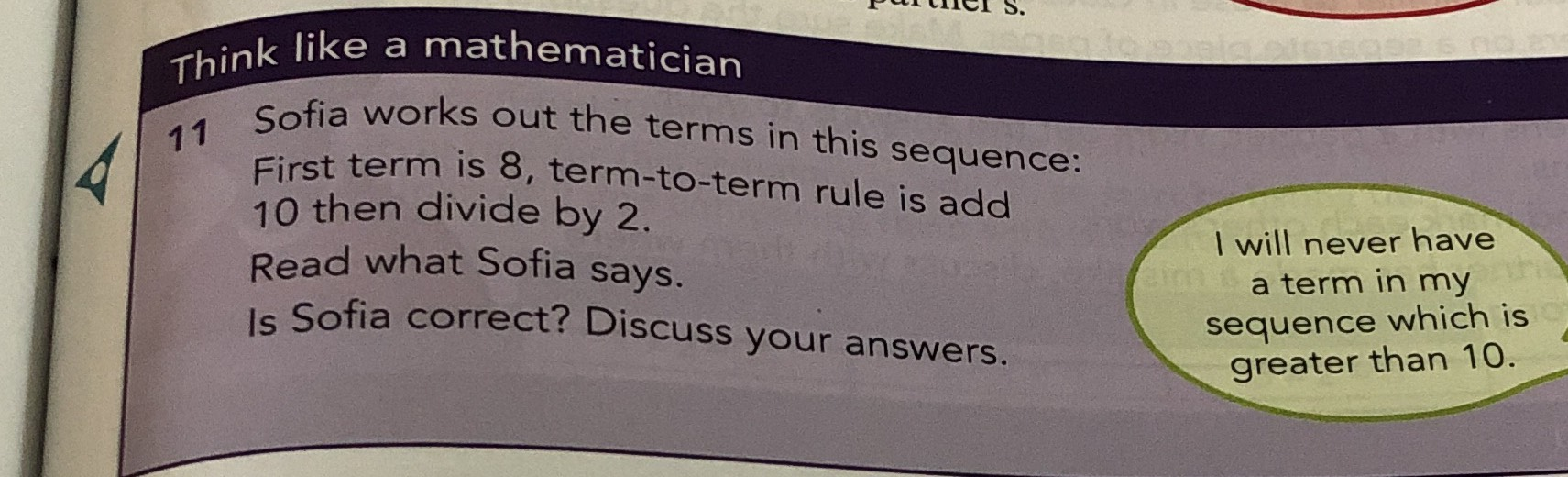### Still have math questions?

Arithmetic
Question$$11$$ Sofia works out the terms in this sequence: First term is $$8$$ , term-to-term rule is add

$$10$$ then divide by $$2$$ . Read what Sofia says. Is Sofia correct? Discuss your answers.